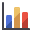The Rocscience International Conference 2021 Proceedings are now available. Read Now

# Histogram Plot

A histogram plot is a bar chart that shows the probability that a variable will have a value in a particular range (bin). The vertical axis is the probability and the horizontal axis is the range of values from minimum to maximum that the variable can take.

To create a Histogram plot:

1. Select Histogram Plotfrom the toolbar or the Statistics menu.
2. From the dialog that appears, select the Variable to Plot and Number of Bins (see below for details).
3. You may also choose the following plotting options using the check boxes provided. See the Plotting Options topic for details.
• Show Input Distribution
• Show Fitted Distribution
• Attach Markers
• Show Failed
4. Click OK to create the histogram.

## Variable to Plot

The user can create a histogram plot for any calculated (output) variable, or any input variable that has a statistical distribution. In the Variable to Plot list, the output variables are always listed first (e.g. Factor of Safety, Tunnel Convergence), followed by the input random variables (e.g. In-Situ Stress, Young's Modulus, etc.)

## Number of Bins

Number of Bins determines the number of intervals between the minimum and maximum values of the variable. The maximum allowed number is 500. Note that if the interval size becomes too small for the program to display, number of bins is automatically reduced to one.